Next: Two View Geometry Up: No Title Previous: Computing Plane Projective

# Summary of Plane Projective Transformations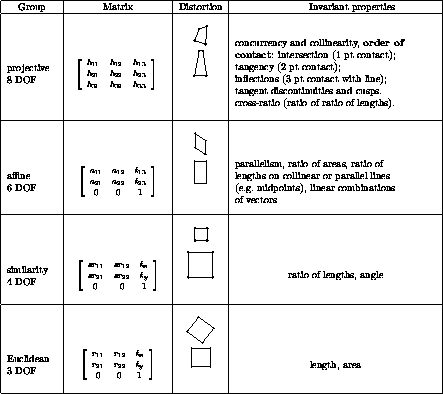Geometric properties invariant to commonly occurring plane transformations. Transformations lower in the table inherit the invariants of those above, but the converse is not true. The matrix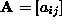is an invertible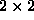matrix,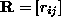is a 2D rotation matrix, and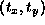a 2D translation. The distortion column shows typical effects of the transformations on a square. Transformations higher in the table can produce all the actions of the ones below. These range from Euclidean, where only translations and rotations occur, to projective where the square can be transformed to any arbitrary quadrilateral (provided no three points are collinear).

Bob Fisher
Wed Apr 16 00:58:54 BST 1997